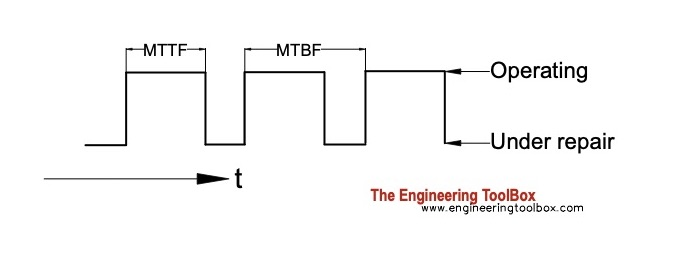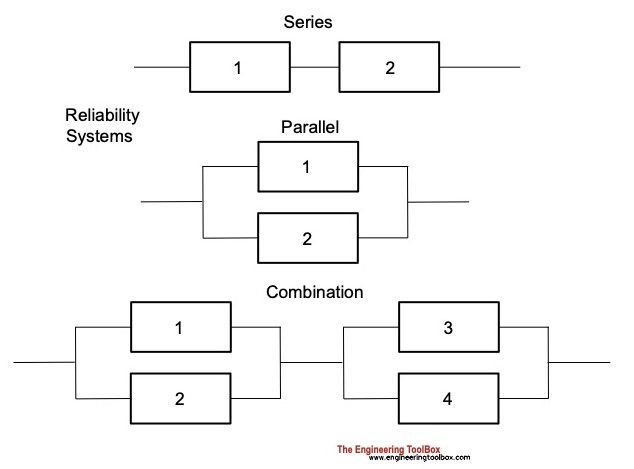Engineering ToolBox - Resources, Tools and Basic Information for Engineering and Design of Technical Applications!

# Reliability of Machine Components

## Mean Time Between Failure - MTB and reliability of machine components and systems.

Reliability characterizes components or system of components by the probability they will perform the desired functions for a given time.

In general -

• more components and/or more complicated systems reduces reliability
• simpler systems with few components increases reliability

Reliability equations:

### Reliability

Reliability at a given time:

R = e-λt                                (1)

where

R = reliability. Values between 0 - 1 where value 1 indicates 100% live components and value 0 indicates 0% live components.

λ = proportional failure rate - a failure rate expressed as a proportion of initial number of live components - No - at time t

t = time (hours) - Note! other units can be used as long as the use is consistent through the calculations.

The failure rate at time t can be expressed as

λ = NF / (No t)

= (No - Ns) / (No t)                 (2)

where

NF = No - Ns = number of failing components at time t

Ns = number of live surviving components at time t

No = initial number of live components at time zero

#### Example - Failure Rate and Reliability

A car manufacturer sells 400000 cars of a certain model in one year. During the the first tree years the owners of 50000 of these cars experience major failures. The failure rate can be calculated as

λ = (50000 cars)/ ((400000 cars) (3 years))

= 0.042 (per year)

The reliability of a model of this car within three years can be calculated as

R = e-(0.042 1/year) (3 year)

= 0.88

= 88 %

### Unreliability - the Probability for a Device to Fail

The connection between reliability and unreliability:

R + Q = 1                              (3)

where

Q = unreliability. Values between 0 - 1 where value 1 indicates 0% live components and value 0 indicates 100% live components.

(1) and (2) can be used to express unreliability

Q = 1 - e-λt                               (4)

#### Example - Unreliability

The unreliability of the car model in the example above within three years can be calculated as

Q = 1 - e-(0.042 1/year) (3 year)

= 0.12

= 12 %

### Number of Live Components

The number of live surviving components in a system at a given time:

Ns = No e-λt                            (5)

### Number of Failure Components

The number of failure dead components in a system at a given time:

Ns = No (1 - e-λt)                        (6)

### Mean Time Between Failures - MTBF

Mean time between failures - MTBF:

MTBF = 1 / λ                         (7)

where

MTBF = Mean Time Between Failure (hours)

• MTTF - Mean Time To Failure is an alternative to MTBF#### Example - Mean Time Between Failure

From the example above the failure rate is 0.42 per year. MTBF can be calculated as

MTBF = 1 / (0.042 1/year)

= 23.8 years

Mean Time Between Failure (MTBF) can be determined by rating Total Surviving Hours against Number of Failures as

MTBF = ts / NF                      (8)

where

ts = total surviving hours (hours)

Combining (5) with the formulas for reliability and more

R = e-t/m                        (1b)

Q = 1 - e-t/m                       (4b)

Ns = No e-t/m                      (5b)

Nf = No (1 - e-t/m)                     (6b)

### MTBF vs. MTTF vs. MTTR

• MTBF - Mean Time Between Failures > commonly used to determine average time between failures
• MTTF - Mean Time To Failure > commonly used for replaceable products that cannot be repaired
• MTTR - Mean Time To Repair > commonly used to determine how long it will take to get a failed product running again

MTBF and MTTF are often used interchangeably.

### Systems Reliability

There are two basic types of reliability systems - series and parallel - and combinations of them.

• in a series system - all devices in the system must work for the system to work
• in a parallel system - the system works if at least one device in the system works#### Reliability of Systems in Series

Reliability of systems in series can be expressed as

Rs = R1 R2                        (9)

where

Rs = system reliability

R1,2 = subsystem reliability

##### Example - Reliability of Systems in Series

From the example above the reliability of a car over three year is 0.88. If we depends on two cars for a mission - whatever it is - the reliability for our mission will be

Rs = 0.88 0.88

= 0.77

= 77 %

#### Reliability of Systems in Parallel

Reliability of systems in parallel can be expressed as

Rs = R1 Q2 + R2 Q1 + R1 R2                       (10)

where

Q1,2 = (1 - R1,2) subsystem unreliability

##### Example - Reliability of Systems in Parallel

From the example above the reliability of a car over three year is 0.88. If it is enough with one of our two cars for our mission - the reliability for our mission can be calculated as

Rs = 0.88 (1- 0.88) + 0.88 (1- 0.88) + 0.88 0.88

= 0.99

= 99 %

Note! - two systems in parallel with 99 % reliability vs. one system alone with reliability 88 % shows the power of redundancy.

## Engineering ToolBox - SketchUp Extension - Online 3D modeling!

Add standard and customized parametric components - like flange beams, lumbers, piping, stairs and more - to your Sketchup model with the Engineering ToolBox - SketchUp Extension - enabled for use with the amazing, fun and free SketchUp Make and SketchUp Pro .Add the Engineering ToolBox extension to your SketchUp from the SketchUp Pro Sketchup Extension Warehouse!

Translate

## Privacy

We don't collect information from our users. Only emails and answers are saved in our archive. Cookies are only used in the browser to improve user experience.

Some of our calculators and applications let you save application data to your local computer. These applications will - due to browser restrictions - send data between your browser and our server. We don't save this data.

## Citation

• Engineering ToolBox, (2005). Reliability of Machine Components. [online] Available at: https://www.engineeringtoolbox.com/reliability-d_953.html [Accessed Day Mo. Year].

Modify access date.

. .

#### Scientific Online Calculator3 30